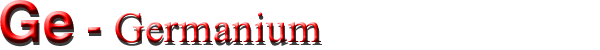Electrical properties

Basic Parameters
Mobility and Hall Effect
Transport Properties in High Electric Fields
Impact Ionization
Recombination Parameters

Basic Parameters

 Breakdown field ≈105V cm-1 Mobility electrons ≤3900 cm2 V-1s-1 Mobility holes ≤1900 cm2 V-1s-1 Diffusion coefficient electrons ≤100 cm2 s-1 Diffusion coefficient holes ≤50 cm2 s-1 Electron thermal velocity 3.1·105m s-1 Hole thermal velocity 1.9·105m s-1

Mobility and Hall Effect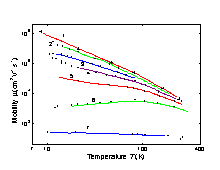Electron mobility versus temperature for different doping levels. 1. High purity Ge; time-of-flight technique (Jacoboni et al. ); 2-6. Hall effect Nd - Na(cm-3): 2. 1·1013; 3. 1.4·1014; 4. 1.7·1015; 5. 7.5·1015; 6. 5.5·1016 (Debye and Conwell ); 7. Hall effect Nd - Na=1.2·1019(cm-3) (Fistul et al. ).
For weakly doped Ge in the range 77-300 K electron mobility
µn≈ 4.9·107·T-1.66 (cm2 V-1 s-1).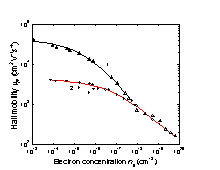Electron Hall mobility versus electron concentration 1. T = 77 K; 2. T = 300 K. (Fistul et al. ).

Approximate formula for the Hall mobility. 300 K.

µnOH/(1+Nd·10-17)1/2,
where µOH≈=3900 (cm2 V-1 s-1),
Nd- in cm-3
(Hilsum ).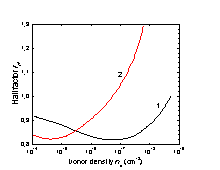The electron Hall factor versus donor density. 1. T = 300 K; 2. T = 77 K. (Babich et al. ).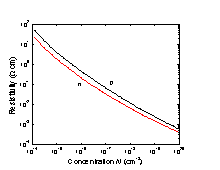Resistivity versus impurity concentration., T = 300 K. (Cuttris ).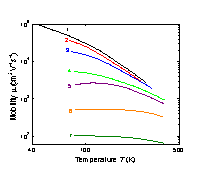Temperature dependences of hole mobility for different doping levels. 1. High purity Ge; time-of-flight technique (Ottaviany et al. ). 2-7. Hall-effect (Golikova et al. ). Na- Nd (cm-3): 2. = 4.9·1013; 3. 3.2·1015; 4. 2.7·1016; 5. 1.2·1017; 6. 4.9·1018; 7. 2.0·1020.
For weakly doped Ge in the range 100-300 K hole mobility
µp≈1.05·109·T-2.33 (cm2 V-1 s-1)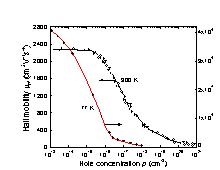The hole Hall mobility versus hole concentration. Experimental points: data from three References (Golikova et al. ).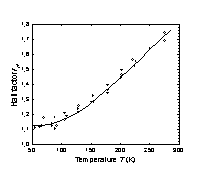The hole Hall factor versus temperature for high purity p-Ge (Morin ).

Transport Properties in High Electric Fields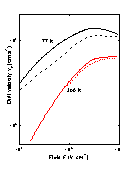Field dependences of the electron drift velocity. Solid lines: F||(100) Solid lines: F||(111). (Jacoboni et al. ).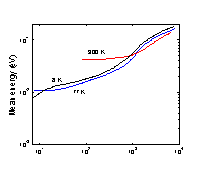Mean energy of electrons in lower valleys as a function of electronic field for three lattice temperatures. (Jacoboni et al. ). (Jacoboni et al. ).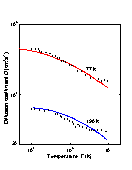The field dependence of longitudinal electron diffusion coefficient D for 77 K and 190 K. F||(100). Solid lines show the results calculation. Symbols represent measured data. (Jacoboni et al. ).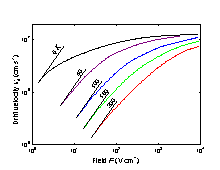Field dependences of the electron drift velocity at different temperatures. F||(100). (Ottaviani et al. ).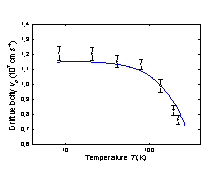Drift velocity vd as a function of temperature for electric field F=104(V cm-1). F||(100). Solid line show the results of calculation in the case where non-parabolic effect are taken into account (Reggiani et al. ).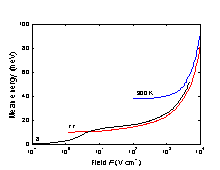Mean energy of hole as a function of electronic field F at different lattice temperatures. Solid line are Monte-Carlo calculations for F||(111) (Reggiani et al. ). Points show experimental results for 82 K. (Vorob'ev et al. ).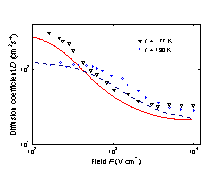The field dependence of longitudinal hole diffusion coefficient D for 77 K and 190 K. F||(111). Dashed and solid lines show the results of the calculations. Symbols represent measured data. (Reggiani et al. ).

Impact Ionization

There are two schools of thought regarding the impact ionization in Ge.

The first one states that impact ionization rates αi and βi for electrons and holes in Ge are known accurately enough to distinguish such subtle details as the anisothropy of αi and βi for different crystallographic directions.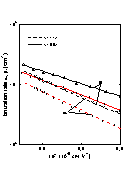Ionization rates in (111) and (100) directions versus 1/F. T = 300 K. (Mikava et al. ).

From (Mikava et al. )

 For electrons: αi = αoexp(-Fno/F) (111) direction αo = 2.72·106 cm-1 Fno = 1.1·106 V cm-1 (100) direction αo = 8.04·106 cm-1 Fno = 1.4·106 V cm-1 For holes: βi = βoexp(-Fpo/F) (111) direction βo = 1.72·106 cm-1 Fpo = 9.37·105 V cm-1 (100) direction βo = 6.39·106 cm-1 Fpo = 1.27·106 V cm-1

The second school contends that the values of αi and βi for the same electric field reported by different researches differ by an order of magnitude. This point of view is explained by Kyuregyan and Yurkov . In accordance with this approach we can assume for all crystallographic directions that
 For electrons: αi=αoexp(-Fno/ F) where αo = 2.84·106 cm-1 Fno = 1.14·106 V cm-1 For holes: βi=βoexp(-Fpo/ F) where βo = 4.21·106 cm-1 Fpo = 1.11·106 V cm-1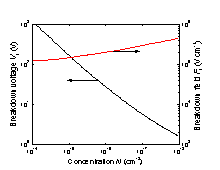Breakdown voltage and breakdown field versus doping density for an abrupt p-n junction. (Kyuregyan and Yurkov ).

Recombination Parameters

 Pure n-type material 300 K The longest lifetime of holes τp≥ 10-3 s Diffusion length Lp≥ 0.2 cm 77 K The longest lifetime of holes τp≥ 10-4 s Diffusion length Lp≥ 0.15 cm Pure p-type material 300 K The longest lifetime of electrons τn≥ 10-3 s Diffusion length Ln≥ 0.3 cm 77 K The longest lifetime of electrons τn≥ 10-4 s Diffusion length Ln≥ 0.15 cm Surface recombination 10 ÷ 106cm/s. Radiative recombination coefficient at 300 K 6.41·10-14 cm3 s-1 Auger coefficient at 300 K ~10-30 cm6 s-1